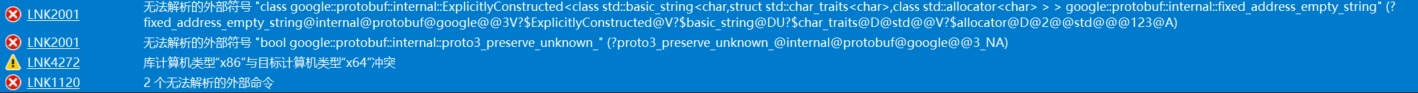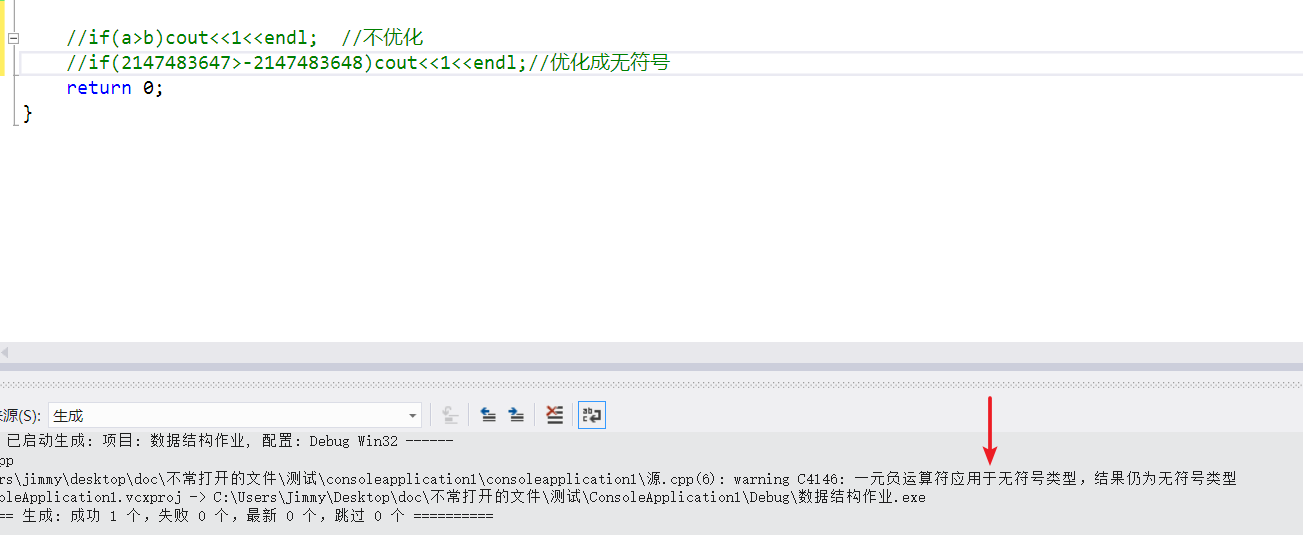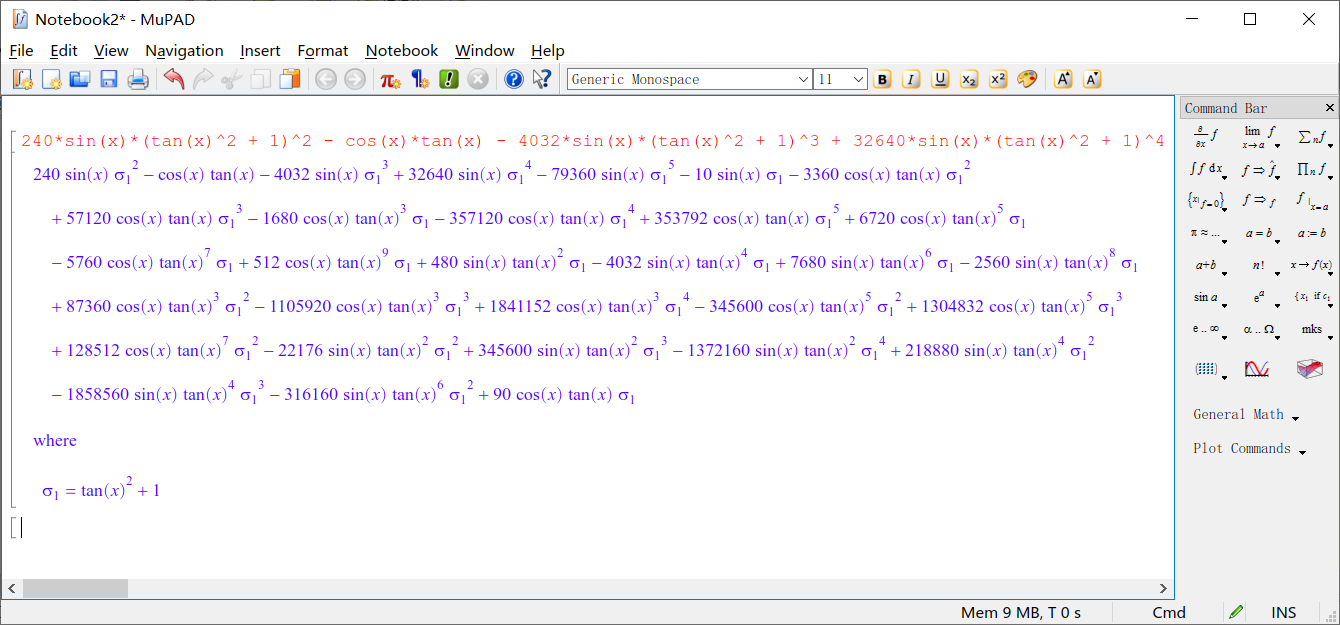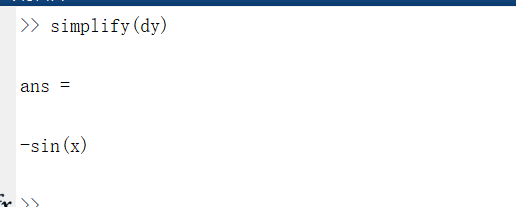• 一元负运算符应用于无符号类型，从而导致无符号结果。 C4308 /we4308 一个负整型常数转换为无符号类型，从而导致一个可能无意义结果。 C4532 /we4532 __finally /finally中的关键词，使用continue， ...
查看MSDN中对SDL的描述，SDL检查会视以下告警为错误：

/sdl 启用警告

等效的命令行开关

描述

C4146

/we4146

一元负运算符应用于无符号类型，从而导致无符号结果。

C4308

/we4308

一个负整型常数转换为无符号类型，从而导致一个可能无意义结果。

C4532

/we4532

__finally /finally中的关键词，使用continue， break 或 goto在异常终止块未定义行为。

C4533

/we4533

初始化变量的代码不会执行。

C4700

/we4700

使用未初始化的局部变量。

C4703

/we4703

对一个潜在的未初始化的局部指针变量的使用。

C4789

/we4789

当使用时，请缓冲区溢出特定 C 运行时 (CRT) 函数。

C4995

/we4995

使用函数的标deprecated。

C4996

/we4996

使用函数的标记作为deprecated。
这里个问题在预编译中加上宏PROTOBUF_USE_DLLS就可以搞定了展开全文• c语言的一元运算符号*的作用
这是我第一次在csdn上面写博客。说实话有些胆怯。做了四年的javaweb开发之后，忽然想到要复习一下大学时候学习的c语言。几经周折之后，终于搞明白学习c语言的真正教材是c程序设计语言。真是好奇害死猫啊，我忐忑的下载了这本教材的电子版。说了这么多的废话，我自己都不好意思了。说了这么多，无非是想表达，我找到了一本适合学习c开发的武功秘籍。现在就步入正题吧。其实也没有什么，就是一种顿悟。当我浏览到第五章第一节的时候，有句话让我很兴奋，真的。我看的是翻译版，所以译文是这样的：一元运算符*是间接寻址或间接引用运算符。当她它作用于指针时，将访问指针所指向的对象。读完这句话的时候，我突然联想到汇编语言里面的寻址概念，当时也是稀里糊涂就过去了，没有深入理解其中的意思，现在终于明白了，寻址就是按照地址去寻找地址里面包含的信息，至于是直接还是间接，暂时不讨论了，涉及到汇编的寻址方式了。说了一堆没用的，就是为了表达对*运算符的理解。也许你讲我笨吧，连这个都不明白。不论怎么样我都认了。反正我现在怀着一个空杯的心态再次来学习c语言，主要是大学时候学的太烂，现在想弥补一下逝去的遗憾。

展开全文• warning C4146: 一元负运算符应用于无符号类型，结果仍为无符号类型 当变量的值处于临界状态时会出现该警告warning C4146: 一元负运算符应用于无符号类型，结果仍为无符号类型

当变量的值处于临界状态时会出现该警告


展开全文C
• 文章目录MATLAB符号函数的求导1、符号函数求导1.1 一元符号函数求导1.2 多元函数的导数2、差分2.1 向量的差分2.2 矩阵的差分 MATLAB符号函数的求导 MATLAB中可以使用命令diff对符号函数进行求导，并且当diff作用到...
声明：本文章中数据来自清风老师数学建模课程
文章目录MATLAB符号函数的求导1、符号函数求导1.1 一元符号函数求导1.2 多元函数的导数2、差分2.1 向量的差分2.2 矩阵的差分
MATLAB符号函数的求导
MATLAB中可以使用命令diff对符号函数进行求导，并且当diff作用到向量或者是矩阵时还可以求差分
1、符号函数求导
1.1 一元符号函数求导
先定义一元符号函数
syms x
y = x^4-5*x^2+6

进行求导，当diff作用到符号函数上时可以对符号函数进行求导

第一个参数传入符号函数
第二个参数传入要求几阶导，第二个参数空着默认求1一阶导

diff(y,1) %求一阶导数  diff(y)默认求一阶导
% 4*x^3 - 10*x
diff(y,2) %求二阶导数
% 12*x^2 - 10

求导后分析结果遇到的问题
下面我们用diff对cos(x)*tan(x)进行求导
syms x
y = cos(x)*tan(x)
dy = diff(y,10)  %求十阶导数

240*sin(x)*(tan(x)^2 + 1)^2 - cos(x)*tan(x) - 4032*sin(x)*(tan(x)^2 + 1)^3 + 32640*sin(x)*(tan(x)^2 + 1)^4 - 79360*sin(x)*(tan(x)^2 + 1)^5 - 10*sin(x)*(tan(x)^2 + 1) - 3360*cos(x)*tan(x)*(tan(x)^2 + 1)^2 + 57120*cos(x)*tan(x)*(tan(x)^2 + 1)^3 - 1680*cos(x)*tan(x)^3*(tan(x)^2 + 1) - 357120*cos(x)*tan(x)*(tan(x)^2 + 1)^4 + 353792*cos(x)*tan(x)*(tan(x)^2 + 1)^5 + 6720*cos(x)*tan(x)^5*(tan(x)^2 + 1) - 5760*cos(x)*tan(x)^7*(tan(x)^2 + 1) + 512*cos(x)*tan(x)^9*(tan(x)^2 + 1) + 480*sin(x)*tan(x)^2*(tan(x)^2 + 1) - 4032*sin(x)*tan(x)^4*(tan(x)^2 + 1) + 7680*sin(x)*tan(x)^6*(tan(x)^2 + 1) - 2560*sin(x)*tan(x)^8*(tan(x)^2 + 1) + 87360*cos(x)*tan(x)^3*(tan(x)^2 + 1)^2 - 1105920*cos(x)*tan(x)^3*(tan(x)^2 + 1)^3 + 1841152*cos(x)*tan(x)^3*(tan(x)^2 + 1)^4 - 345600*cos(x)*tan(x)^5*(tan(x)^2 + 1)^2 + 1304832*cos(x)*tan(x)^5*(tan(x)^2 + 1)^3 + 128512*cos(x)*tan(x)^7*(tan(x)^2 + 1)^2 - 22176*sin(x)*tan(x)^2*(tan(x)^2 + 1)^2 + 345600*sin(x)*tan(x)^2*(tan(x)^2 + 1)^3 - 1372160*sin(x)*tan(x)^2*(tan(x)^2 + 1)^4 + 218880*sin(x)*tan(x)^4*(tan(x)^2 + 1)^2 - 1858560*sin(x)*tan(x)^4*(tan(x)^2 + 1)^3 - 316160*sin(x)*tan(x)^6*(tan(x)^2 + 1)^2 + 90*cos(x)*tan(x)*(tan(x)^2 + 1)

然而并不理想，见下图：这时候我们可以尝试一下simplify来进行化简整理
如下图所示，结果很明显，是我们想要的形式那么为什么我们使用diff对cos(x)*tan(x)求导的结果这么复杂呢，其实它的求导算法就是莱布尼兹公式的展开。其实我们知道cos(x)*tan(x)等于sin(x),求高次导数一定是一个简单的式子

1.2 多元函数的导数
参数：

第一个参数是待求导符号函数
第二个参数是要对那个自变量求偏导
第三个参数是要求几阶偏导数

当连续对自变量求偏导时，放在前面的自变量先求偏导，后面的自变量后求偏导
syms x1 x2 x3
y1 = x1^5*x2+x2*x3-x1^2*x3
py1 = diff(y1,x1,1) % 对x1求一阶偏导
% 5*x2*x1^4 - 2*x3*x1
py2 = diff(y1,x1,2) % 对x1求二阶偏导
% 20*x2*x1^3 - 2*x3
py3 = diff(y1,x1,x2) % 先对x1求偏导，再对x2求偏导
% 5*x1^4
py4 = diff(y1,x2,x1) % 先对x2求偏导，再对x1求偏导
% 5*x1^4

2、差分
注意，如果diff函数作用的对象不是符号函数，而是矩阵，那么对应的功能是求差分。
2.1 向量的差分
默认求1阶差分
A=[4 5 6 3 2 1];
diff(A)  % 求向量A的一阶差分     1     1    -3    -1    -1
diff(A,2)  % 在一阶差分的基础上再差分一次     0    -4     2     0

2.2 矩阵的差分
默认是列的方向上求差分（下一行减上一行），且默认求一阶差分。
第三个参数指定为2时，在行的方向上求差分（后一列减前一列）
第二个参数用于指定求几阶差分，默认一阶差分
A=[4 5 6;
7 4 2;
5 6 2]
A1=diff(A)  % 下一行减去上一行求一阶差分
%      3    -1    -4
%     -2     2     0
A2=diff(A,2) % 下一行减去上一行求二阶差分（在一阶差分的基础上再差分一次）
%     -5     3     4
A3=diff(A,2,1) % 最后面的1表示在行上进行差分（在列的方向上进行差分）
%     -5     3     4
A4=diff(A,1,2)  % 后一列减去前一列求一阶差分， 最后面的2表示在列上进行差分（在行的方向上进行差分）
%      1     1
%     -3    -2
%      1    -4
A4=diff(A,2,2) % 后一列减去前一列求二阶差分
%      0
%      1
%     -5




展开全文matlab
• VS错误解决 C4146 一元负运算符应用于无符号类型 结果仍为无符号类型 在VS2015下编译NTL库的时候，我编译了NTL库源代码生成静态链接库，在应用程序设置那一步，虽然将应用程序选成了静态链接库，也没有勾选预编译头...
• VS编译protobuf时，出现error C4146: 一元负运算符应用于无符号类型，结果仍为无符号类型。 解决办法——关闭SDL检查 右键选中要配置的属性的项目->配置属性->C/C+±>SDL检查，选择否，如下图所示 ...
• 单组设计一元定量资料 t检验 与 符号秩和检验 1.1.1 问题与数据 例1-1 已知玉米单交种群 105 的平均穗重为300克。喷药后，随机抽取九个果穗。其穗重分别为308g、305g、298g、315g、300g、321g、294g、320g。 问：...数据分析
• error C4146: 一元负运算符应用于无符号类型，结果仍为无符号类型 warning C4244: “=”: 从“double”转换到“float”，可能丢失数据 error C4146: 一元负运算符应用于无符号类型，结果仍为无符号类型 ...VS2015
• 在二元运算的基础上，引入整数运算和一元符号运算。具体博文参见http://u.bb/355623/1425arithmetic-expressions
• error C4146: 一元负运算符应用于无符号类型，结果仍为无符号类型 原因： 由于编译器SDL安全检查认为这一操作（通常是为无符号整形取负的操作）无效而产生的。 这里我们假设定义一个数值为int INT，在32位机上面...
• “-2147483648 ”按无符号数比较， 10……0B比01……1B大，结果为false。 在ISO C99标准下 ，2147483648为long long型，因此 “-2147483648 ”按带符号整数比较， 10……0B比01……1B小，结果为true。
• 在我把gmp.h .lib 和.dll文件在vs2017中配置好之后，我找了段gmp代码来测试,结果会出现这个问题：error C4146: 一元负运算符应用于无符号类型，结果仍为无符号类型  我的代码如下： #include &lt;gmp.h&...
• 在使用VS2015编写程序时候遇到这样的问题，一样的代码在LINUX下却...VS2015中一元负运算符应用于无符号类型的原因和解决 原因： 这一问题是由于编译器SDL安全检查认为这一操作（通常是为无符号整形取负的操作）无...
• (defun space-over (n) (cond (() (format t "Error!~%")) ((zerop n) nil) (t (or (format t " ") (space-over (- n 1)))))) (defun plot-one-point (plotting-string y-val) (space-over y-val)
• c:\workspace\test\test\gmp.h(2177): error C4146: 一元负运算符应用于无符号类型，结果仍为无符号类型。。。这个怎么解决呢。 gmp.h文件中报错部分代码。。。 #if defined (__GMP_EXTERN_INLINE) || ......